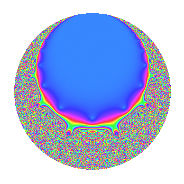Properties

 Label 8.18.a.aLevel 8 Weight 18 Character orbit 8.a Self dual Yes Analytic conductor 14.658 Analytic rank 0 Dimension 2 CM No Inner twists 1

Related objects

Newspace parameters

 Level: $$N$$ = $$8 = 2^{3}$$ Weight: $$k$$ = $$18$$ Character orbit: $$[\chi]$$ = 8.a (trivial)

Newform invariants

 Self dual: Yes Analytic conductor: $$14.6577669876$$ Analytic rank: $$0$$ Dimension: $$2$$ Coefficient field: $$\Q(\sqrt{2146})$$ Coefficient ring: $$\Z[a_1, a_2, a_3]$$ Coefficient ring index: $$2^{8}$$ Fricke sign: $$-1$$ Sato-Tate group: $\mathrm{SU}(2)$

$q$-expansion

Coefficients of the $$q$$-expansion are expressed in terms of $$\beta = 256\sqrt{2146}$$. We also show the integral $$q$$-expansion of the trace form.

 $$f(q)$$ $$=$$ $$q$$ $$+ ( -476 + \beta ) q^{3}$$ $$+ ( -26810 + 60 \beta ) q^{5}$$ $$+ ( -166584 + 978 \beta ) q^{7}$$ $$+ ( 11726669 - 952 \beta ) q^{9}$$ $$+O(q^{10})$$ $$q$$ $$+ ( -476 + \beta ) q^{3}$$ $$+ ( -26810 + 60 \beta ) q^{5}$$ $$+ ( -166584 + 978 \beta ) q^{7}$$ $$+ ( 11726669 - 952 \beta ) q^{9}$$ $$+ ( 215487340 - 74781 \beta ) q^{11}$$ $$+ ( 1333760974 + 329628 \beta ) q^{13}$$ $$+ ( 8451176920 - 55370 \beta ) q^{15}$$ $$+ ( 30336751634 - 1629816 \beta ) q^{17}$$ $$+ ( 89314980020 + 1134309 \beta ) q^{19}$$ $$+ ( 137625464352 - 632112 \beta ) q^{21}$$ $$+ ( 264378297304 + 18242742 \beta ) q^{23}$$ $$+ ( -255915755425 - 3217200 \beta ) q^{25}$$ $$+ ( -78000700568 - 116960342 \beta ) q^{27}$$ $$+ ( -3620330045730 + 71618988 \beta ) q^{29}$$ $$+ ( -939175570144 + 165125256 \beta ) q^{31}$$ $$+ ( -10619790957776 + 251083096 \beta ) q^{33}$$ $$+ ( 8257236339120 - 36215220 \beta ) q^{35}$$ $$+ ( -10166232283290 - 2527244916 \beta ) q^{37}$$ $$+ ( 45724096081144 + 1176858046 \beta ) q^{39}$$ $$+ ( -881520952662 + 4750902096 \beta ) q^{41}$$ $$+ ( 96697262984332 - 1772936949 \beta ) q^{43}$$ $$+ ( -8347763418610 + 729123260 \beta ) q^{45}$$ $$+ ( 50381918882736 - 12557431380 \beta ) q^{47}$$ $$+ ( -98082609138247 - 325838304 \beta ) q^{49}$$ $$+ ( -243658033250680 + 31112544050 \beta ) q^{51}$$ $$+ ( -158909073030026 - 40341828660 \beta ) q^{53}$$ $$+ ( -636810354621560 + 14934119010 \beta ) q^{55}$$ $$+ ( 117015577653584 + 88775048936 \beta ) q^{57}$$ $$+ ( -631025394160868 - 86330682801 \beta ) q^{59}$$ $$+ ( 1382929846361854 - 97871720196 \beta ) q^{61}$$ $$+ ( -132897429619032 + 11627270250 \beta ) q^{63}$$ $$+ ( 2745779846573140 + 71188331760 \beta ) q^{65}$$ $$+ ( 981503272275044 + 62855476593 \beta ) q^{67}$$ $$+ ( 2439819835505248 + 255694752112 \beta ) q^{69}$$ $$+ ( 241819553552264 + 82368492066 \beta ) q^{71}$$ $$+ ( -1088446174295606 - 741325171608 \beta ) q^{73}$$ $$+ ( -330651932020900 - 254384368225 \beta ) q^{75}$$ $$+ ( -10321736909335968 + 223203936624 \beta ) q^{77}$$ $$+ ( -2647919952813872 + 468379685172 \beta ) q^{79}$$ $$+ ( -17926588053364231 + 100613857400 \beta ) q^{81}$$ $$+ ( 4986259009443572 + 631666459029 \beta ) q^{83}$$ $$+ ( -14566392679681300 + 1863900465000 \beta ) q^{85}$$ $$+ ( 11795789908548408 - 3654420684018 \beta ) q^{87}$$ $$+ ( -355549518406950 - 1713360438936 \beta ) q^{89}$$ $$+ ( 45116885807970288 + 1249507481820 \beta ) q^{91}$$ $$+ ( 23670305847294080 - 1017775192000 \beta ) q^{93}$$ $$+ ( 7177235874250040 + 5328487976910 \beta ) q^{95}$$ $$+ ( 57435273304985954 - 48030348120 \beta ) q^{97}$$ $$+ ( 12539341182577532 - 1082075982169 \beta ) q^{99}$$ $$+O(q^{100})$$ $$\operatorname{Tr}(f)(q)$$ $$=$$ $$2q$$ $$\mathstrut -\mathstrut 952q^{3}$$ $$\mathstrut -\mathstrut 53620q^{5}$$ $$\mathstrut -\mathstrut 333168q^{7}$$ $$\mathstrut +\mathstrut 23453338q^{9}$$ $$\mathstrut +\mathstrut O(q^{10})$$ $$2q$$ $$\mathstrut -\mathstrut 952q^{3}$$ $$\mathstrut -\mathstrut 53620q^{5}$$ $$\mathstrut -\mathstrut 333168q^{7}$$ $$\mathstrut +\mathstrut 23453338q^{9}$$ $$\mathstrut +\mathstrut 430974680q^{11}$$ $$\mathstrut +\mathstrut 2667521948q^{13}$$ $$\mathstrut +\mathstrut 16902353840q^{15}$$ $$\mathstrut +\mathstrut 60673503268q^{17}$$ $$\mathstrut +\mathstrut 178629960040q^{19}$$ $$\mathstrut +\mathstrut 275250928704q^{21}$$ $$\mathstrut +\mathstrut 528756594608q^{23}$$ $$\mathstrut -\mathstrut 511831510850q^{25}$$ $$\mathstrut -\mathstrut 156001401136q^{27}$$ $$\mathstrut -\mathstrut 7240660091460q^{29}$$ $$\mathstrut -\mathstrut 1878351140288q^{31}$$ $$\mathstrut -\mathstrut 21239581915552q^{33}$$ $$\mathstrut +\mathstrut 16514472678240q^{35}$$ $$\mathstrut -\mathstrut 20332464566580q^{37}$$ $$\mathstrut +\mathstrut 91448192162288q^{39}$$ $$\mathstrut -\mathstrut 1763041905324q^{41}$$ $$\mathstrut +\mathstrut 193394525968664q^{43}$$ $$\mathstrut -\mathstrut 16695526837220q^{45}$$ $$\mathstrut +\mathstrut 100763837765472q^{47}$$ $$\mathstrut -\mathstrut 196165218276494q^{49}$$ $$\mathstrut -\mathstrut 487316066501360q^{51}$$ $$\mathstrut -\mathstrut 317818146060052q^{53}$$ $$\mathstrut -\mathstrut 1273620709243120q^{55}$$ $$\mathstrut +\mathstrut 234031155307168q^{57}$$ $$\mathstrut -\mathstrut 1262050788321736q^{59}$$ $$\mathstrut +\mathstrut 2765859692723708q^{61}$$ $$\mathstrut -\mathstrut 265794859238064q^{63}$$ $$\mathstrut +\mathstrut 5491559693146280q^{65}$$ $$\mathstrut +\mathstrut 1963006544550088q^{67}$$ $$\mathstrut +\mathstrut 4879639671010496q^{69}$$ $$\mathstrut +\mathstrut 483639107104528q^{71}$$ $$\mathstrut -\mathstrut 2176892348591212q^{73}$$ $$\mathstrut -\mathstrut 661303864041800q^{75}$$ $$\mathstrut -\mathstrut 20643473818671936q^{77}$$ $$\mathstrut -\mathstrut 5295839905627744q^{79}$$ $$\mathstrut -\mathstrut 35853176106728462q^{81}$$ $$\mathstrut +\mathstrut 9972518018887144q^{83}$$ $$\mathstrut -\mathstrut 29132785359362600q^{85}$$ $$\mathstrut +\mathstrut 23591579817096816q^{87}$$ $$\mathstrut -\mathstrut 711099036813900q^{89}$$ $$\mathstrut +\mathstrut 90233771615940576q^{91}$$ $$\mathstrut +\mathstrut 47340611694588160q^{93}$$ $$\mathstrut +\mathstrut 14354471748500080q^{95}$$ $$\mathstrut +\mathstrut 114870546609971908q^{97}$$ $$\mathstrut +\mathstrut 25078682365155064q^{99}$$ $$\mathstrut +\mathstrut O(q^{100})$$

Embeddings

For each embedding $$\iota_m$$ of the coefficient field, the values $$\iota_m(a_n)$$ are shown below.

For more information on an embedded modular form you can click on its label.

Label $$\iota_m(\nu)$$ $$a_{2}$$ $$a_{3}$$ $$a_{4}$$ $$a_{5}$$ $$a_{6}$$ $$a_{7}$$ $$a_{8}$$ $$a_{9}$$ $$a_{10}$$
1.1
 −46.3249 46.3249
0 −12335.2 0 −738361. 0 −1.17649e7 0 2.30166e7 0
1.2 0 11383.2 0 684741. 0 1.14317e7 0 436725. 0
 $$n$$: e.g. 2-40 or 990-1000 Significant digits: Format: Complex embeddings Normalized embeddings Satake parameters Satake angles

Inner twists

This newform does not admit any (nontrivial) inner twists.

Atkin-Lehner signs

$$p$$ Sign
$$2$$ $$-1$$

Hecke kernels

This newform can be constructed as the kernel of the linear operator $$T_{3}^{2}$$ $$\mathstrut +\mathstrut 952 T_{3}$$ $$\mathstrut -\mathstrut 140413680$$ acting on $$S_{18}^{\mathrm{new}}(\Gamma_0(8))$$.